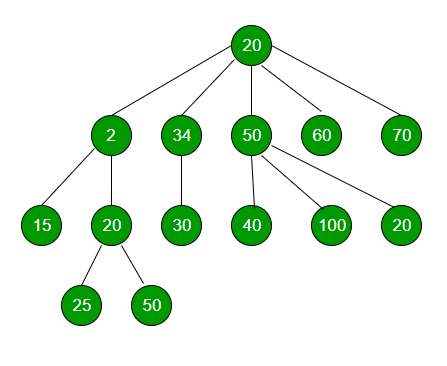# Immediate Smaller element in an N-ary Tree

Given an element x, task is to find the value of its immediate smaller element.Example :

```Input : x = 30 (for above tree)
Output : Immediate smaller element is 25
```

Explanation : Elements 2, 15, 20 and 25 are smaller than x i.e, 30, but 25 is the immediate smaller element and hence the answer.
Approach :

• Let res be the resultant node.
• Initialize the resultant Node as NULL.
• For every Node, check if data of root is greater than res, but less than x. if yes, update res.
• Recursively do the same for all nodes of the given Generic Tree.
• Return res, and res->key would be the immediate smaller element.

Below is the implementation of above approach :

 `// C++ program to find immediate Smaller ` `// Element of a given element in a n-ary tree. ` `#include ` `using` `namespace` `std; ` ` `  `// class of a node of an n-ary tree ` `class` `Node { ` ` `  `public``: ` `    ``int` `key; ` `    ``vector child; ` ` `  `    ``// constructor ` `    ``Node(``int` `data) ` `    ``{ ` `        ``key = data; ` `    ``} ` `}; ` ` `  `// Function to find immediate Smaller Element ` `// of a given number x ` `void` `immediateSmallerElementUtil(Node* root,  ` `                            ``int` `x, Node** res) ` `{ ` `    ``if` `(root == NULL) ` `        ``return``; ` ` `  `    ``// if root is greater than res, but less ` `    ``// than x, then update res ` `    ``if` `(root->key < x) ` `        ``if` `(!(*res) || (*res)->key < root->key) ` `            ``*res = root; ``// Updating res ` ` `  `    ``// Number of children of root ` `    ``int` `numChildren = root->child.size(); ` ` `  `    ``// Recursive calling for every child ` `    ``for` `(``int` `i = 0; i < numChildren; i++) ` `        ``immediateSmallerElementUtil(root->child[i], x, res); ` ` `  `    ``return``; ` `} ` ` `  `// Function to return immediate Smaller ` `// Element of x in tree ` `Node* immediateSmallerElement(Node* root, ``int` `x) ` `{ ` `    ``// resultant node ` `    ``Node* res = NULL; ` ` `  `    ``// calling helper function and using ` `    ``// pass by reference ` `    ``immediateSmallerElementUtil(root, x, &res); ` ` `  `    ``return` `res; ` `} ` ` `  `// Driver program ` `int` `main() ` `{ ` `    ``// Creating a generic tree ` `    ``Node* root = ``new` `Node(20); ` `    ``(root->child).push_back(``new` `Node(2)); ` `    ``(root->child).push_back(``new` `Node(34)); ` `    ``(root->child).push_back(``new` `Node(50)); ` `    ``(root->child).push_back(``new` `Node(60)); ` `    ``(root->child).push_back(``new` `Node(70)); ` `    ``(root->child->child).push_back(``new` `Node(15)); ` `    ``(root->child->child).push_back(``new` `Node(20)); ` `    ``(root->child->child).push_back(``new` `Node(30)); ` `    ``(root->child->child).push_back(``new` `Node(40)); ` `    ``(root->child->child).push_back(``new` `Node(100)); ` `    ``(root->child->child).push_back(``new` `Node(20)); ` `    ``(root->child->child->child).push_back(``new` `Node(25)); ` `    ``(root->child->child->child).push_back(``new` `Node(50)); ` ` `  `    ``int` `x = 30; ` ` `  `    ``cout << ``"Immediate smaller element of "` `<< x << ``" is "``; ` `    ``cout << immediateSmallerElement(root, x)->key << endl; ` ` `  `    ``return` `0; ` `} `

Output :

```Immediate smaller element of 30 is 25
```

Time Complexity : O(N), where N is the number of nodes in N-ary Tree.
Auxiliary Space : O(N), for recursive call(worst case when a node has N number of childs)

Attention reader! Don’t stop learning now. Get hold of all the important DSA concepts with the DSA Self Paced Course at a student-friendly price and become industry ready.

My Personal Notes arrow_drop_upIn love with a semicolon because sometimes i miss it so badly)

If you like GeeksforGeeks and would like to contribute, you can also write an article using contribute.geeksforgeeks.org or mail your article to contribute@geeksforgeeks.org. See your article appearing on the GeeksforGeeks main page and help other Geeks.

Please Improve this article if you find anything incorrect by clicking on the "Improve Article" button below.